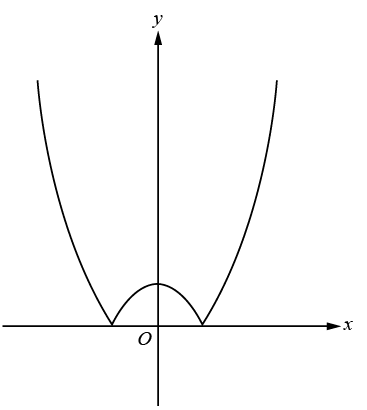# SAT官方每日一题附答案和解析[数学](2015年11月15日)评论打印收藏本文

Mathematics>Standard Multiple ChoiceWhich of the following could be the equation of the function graphed in the xy-plane above?

(A) y = ((-x)^2) + 1
(B) y = -(x^2) + 1
(C) y = |((x^2) + 1)|
(D) y = |((x^2)-1)|
(E) y = |(x-1)^2|

 重点单词 查看全部解释 multiple ['mʌltipl] 想一想再看 adj. 许多，多种多样的n. 倍数，并联 联想记忆 function ['fʌŋkʃən] 想一想再看 n. 功能，函数，职务，重大聚会vi. 运行 option ['ɔpʃən] 想一想再看 n. 选择权，可选物，优先购买权v. 给予选 联想记忆 graph [grɑ:f] 想一想再看 n. 图表，示意图vt. (以图表)表示 contain [kən'tein] 想一想再看 vt. 包含，容纳，克制，抑制vi. 自制 联想记忆 correspond [.kɔris'pɔnd] 想一想再看 vi. 符合，通信，相当 联想记忆 symmetric [si'metrik] 想一想再看 adj. 对称的，匀称的 equation [i'kweiʃən] 想一想再看 n. 相等，方程(式), 等式，均衡 联想记忆 parabola [pə'ræbələ] 想一想再看 n. [数]抛物线
﻿# 可可英语官方微信(微信号:ikekenet)

每天向大家推送短小精悍的英语学习资料.添加方式1.扫描上方可可官方微信二维码。
添加方式2.搜索微信号ikekenet添加即可。